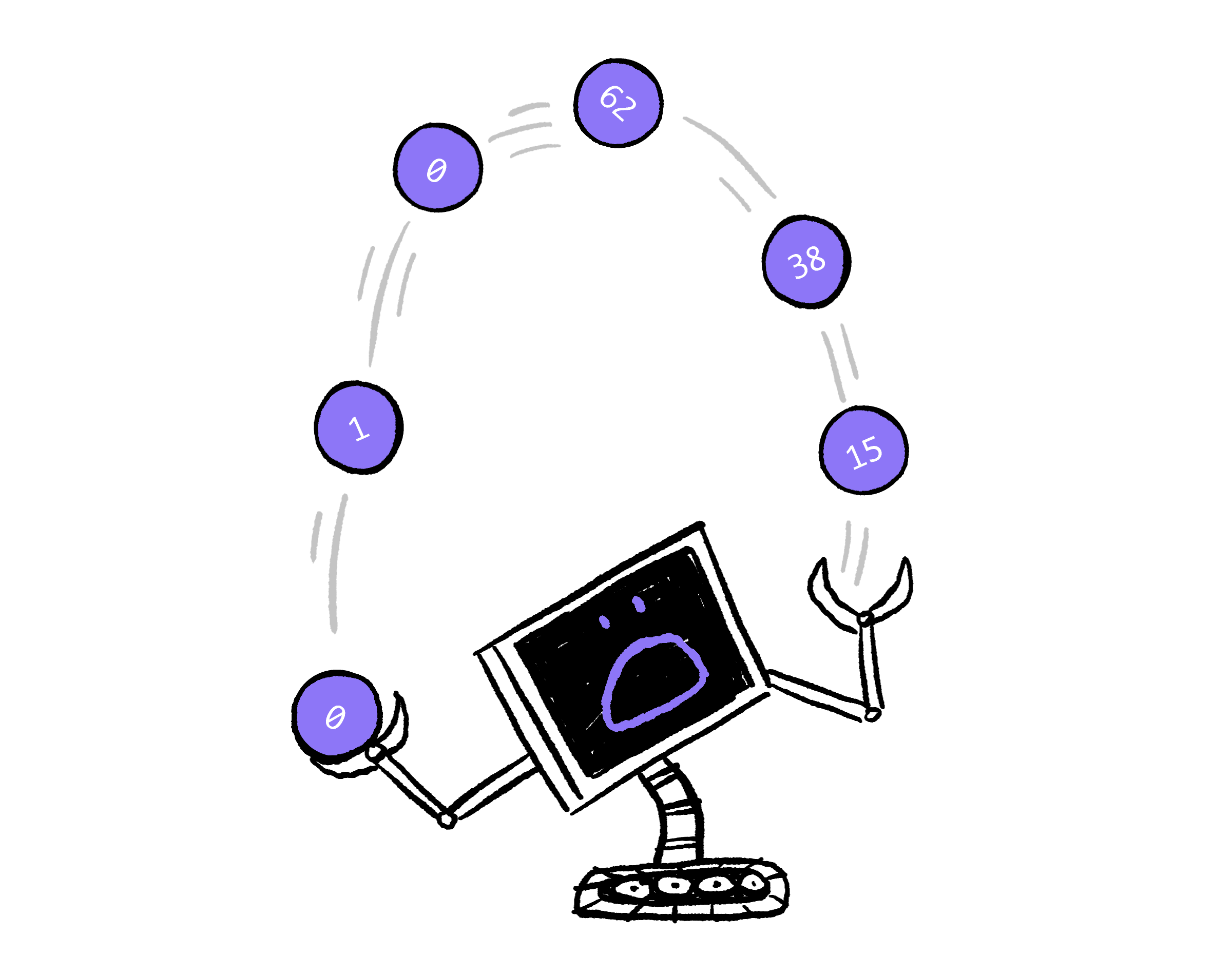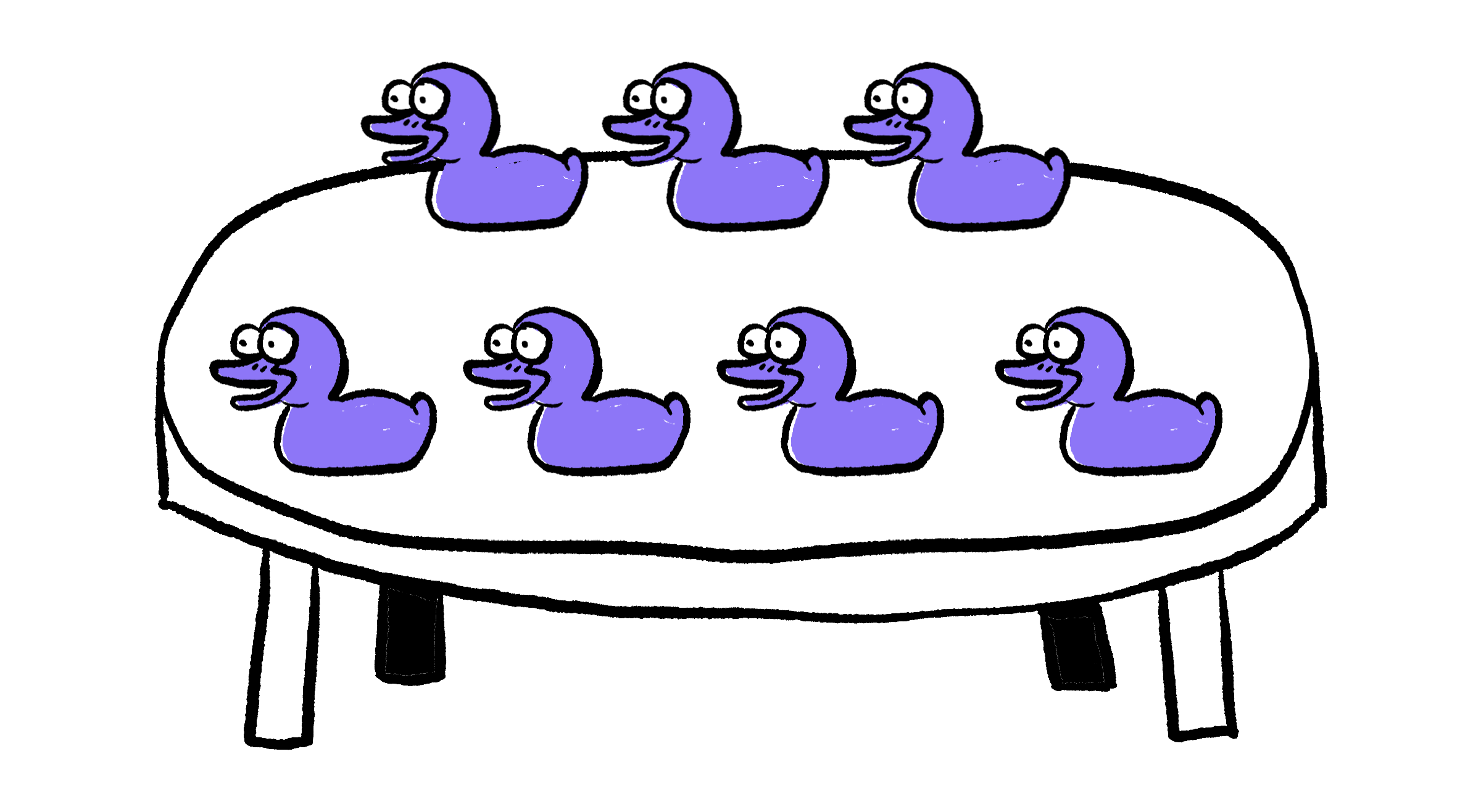### Algorithm Fundamentals

One of the most fundamental things computers do is manipulate numbers. In order to understand the algorithms that computer scientists study, it’s very helpful to understand how algorithms juggle lots of numbers.This quiz will continue to use pseudocode, but the lessons you learn will help you use almost any programming language.

# Manipulating Numbers

The statements 4 < 5, -2 ≤ 5, 8 ≤ 8, 12 > 5, and 4 ≠ 5 are all true, so as tests in a conditional instruction, they will all succeed. On the other hand, 9 ≤ 8, 5 ≠ 5, and 9 = 8 are all false statements. As tests, they will fail.

Will the robot do a dance or sing a song?

# Manipulating Numbers

Numbers need to be stored somewhere in order for algorithms to manipulate them.

This confused sloth princess has four fingers and can have 0, 1, 2, 3, or 4 fingers uncurled."Four."

That makes her hand a place where a number from 0 to 4 can be stored.

A coffee table is another place where a number can be stored.There are seven ducks. Seven.

If you place seven rubber ducks on the table, you can think of the coffee table as storing the number seven. If you put 55 coins on the coffee table, the coffee table is storing the number 55, and so on.

# Manipulating Numbers

Pseudocode, like almost every programming language, has a general notion of places where values live: assignable variables. Assignable variables don’t have much in common with variables from algebra. Like the hand of the confused sloth princess or your coffee table, assignables are places where values can be stored, and possibly replaced by new values.

Sometimes, assignable variables have short, single-letter names. This is just as in algebra when the single letter $x$ is used in the formula $f(x) = x^2 + 2$. It's also common to give assignables more descriptive names, even entire words separated by underscores. Instead of saying “clear any ducks off the table, then put three ducks on the coffee table,” we can write pseudocode with one assignable, ducks_on_table:

# Manipulating Numbers

You just saw a new command that will set the value of an assignable variable to some new value. It's also possible to do arithmetic:

The first thing a set instruction does is to calculate a value from the part of the instruction after the word to. The assignable open_fingers is set to 4 in the first command. Therefore, when the second line is run, ducks_on_table will end up being set to 4 + 3 = 7.

What’s frogs_in_cabinet set to after these commands run?

# Manipulating Numbers

The first thing a set instruction does is to calculate the value of the "right-hand side" — the part after the word to. After that, it sets the assignable variable to that value. This ordering means you can mention the same assignable variable on both sides of a set instruction:

# Manipulating Numbers

Arrange these instructions so that x is set to 4 after all five commands are run. The first command must be either set x to 6 or set x to 2. There are a number of correct answers.

# Manipulating Numbers

One thing that makes algorithms useful general problem-solving tools is that they can have inputs which aren't known ahead of time.

Drag and drop the instructions below to create an algorithm that, regardless of the number n, only announces statements that are true.

For example, if n is $5$, the algorithm should announce "n is positive and odd." If n is $-3.1$, the algorithm has no true statements to announce, so shouldn't run either of the announce instructions.

# Manipulating Numbers

Algorithms will often do arithmetic on inputs, rather than just using them in conditional tests.

Drag and drop the instructions below to build an algorithm where the announce commands only announce the truth, no matter what positive integers x and y are provided as input.

# Manipulating Numbers

You’ve just explored a version of pseudocode with assignable variables, a foundational idea in algorithms and in almost every programming language.

Assignables can hold values (such as numbers), and you've seen some of the kinds of algorithms that can be implemented just with addition, subtraction, and inequality tests between numbers.

To really begin unlocking the power of algorithms, one more tool is needed: repetition.

# Manipulating Numbers

×